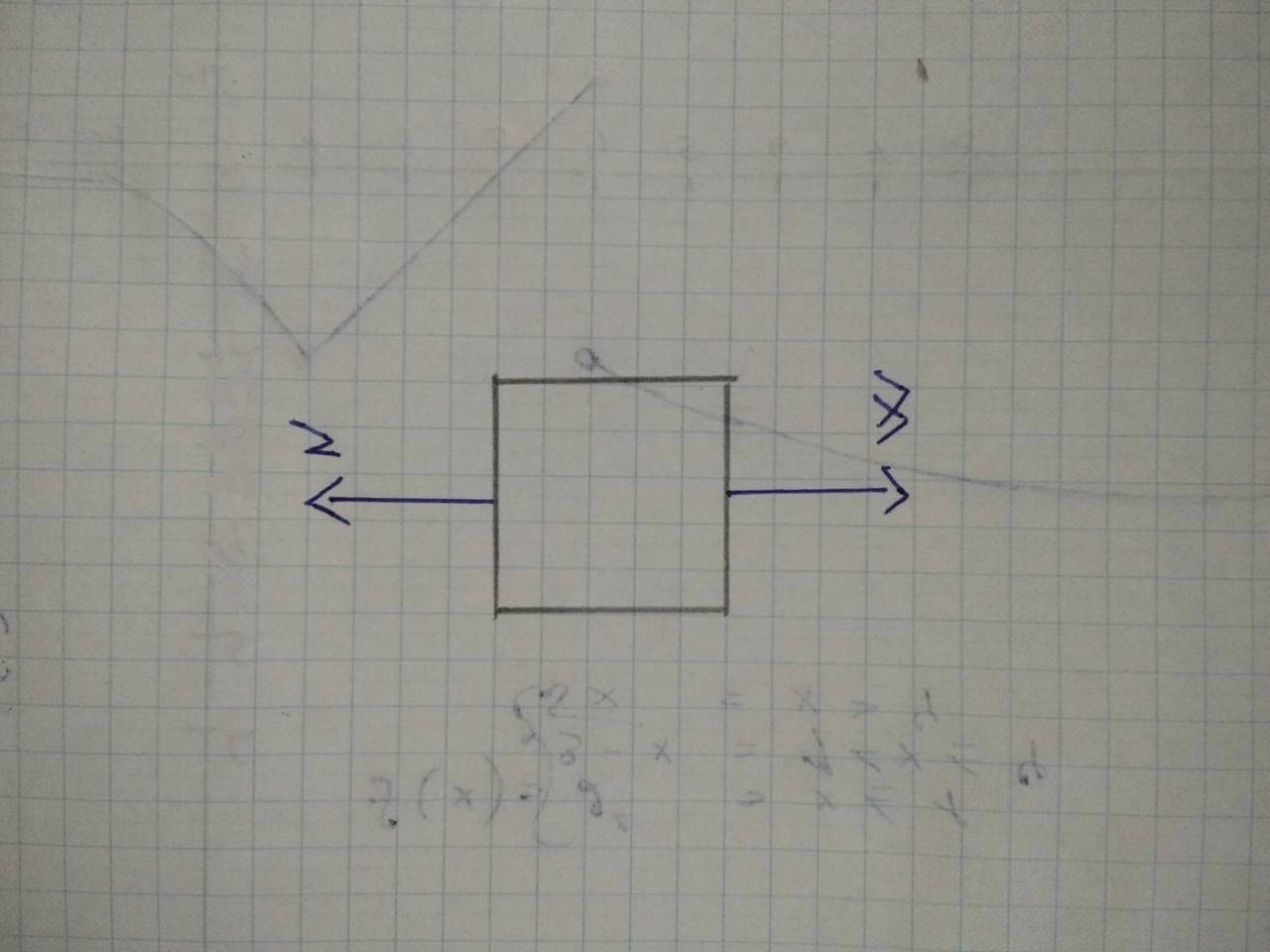A550-N physics student stands on a bathroom scale in an elevator thatJean Blumer 2021-12-20 Answered
A550-N physics student stands on a bathroom scale in an elevator that is supported by a cable. The combined mass of student plus elevator is 850 kg. As the elevator starts moving, the scale reads 450 N.
(a) Find the acceleration of the elevator (magnitude and direction).
(b) What is the acceleration if the scale reads 670 N?
(c) If the scale reads zero, should the student worry? Explain.
(d) What is the tension in the cable in parts (a) and (с)?

• Questions are typically answered in as fast as 30 minutes

Solve your problem for the price of one coffee

• Math expert for every subject
• Pay only if we can solve itstomachdm

Let the +y-axis be upward. The free-body diagrams for the elevator plus student and the student alone are shown in the figure below. The total mass of student plus elevator is $$\displaystyle{M}={850}{k}{g}$$. The tension in the cable is denoted by T.
The normal force exerted by the scale on the student is denoted by n. By Newton's third law, this force is equal in magnitude to the downward pressure exerted by the student's feet on the scale (the scale reading), that is n represents the scale’s reading.
The mass of the student alone, whose actual weight is $$\displaystyle{W}_{{{s}}}={550}{N},{i}{s}{m}_{{{s}}}=\frac{{W}_{{{s}}}}{{g}}={550}\frac{{N}}{{9.80}}\frac{{m}}{{s}^{{{2}}}}={56.1}{k}{g}$$
(a)Application of the y-equation of Newton's second law, $$\displaystyle{E}{F}_{{{y}}}={m}{a}_{{{y}}}$$ gives
$$\displaystyle{n}-{m}_{{{s}}}{g}={m}_{{{s}}}{a}_{{{y}}}.$$
The scale’s reading corresponds to $$\displaystyle{n}={450}{N}$$. Solving for dy, we find
$$\displaystyle{a}_{{{y}}}={\frac{{{n}-{m}_{{{s}}}{g}}}{{{m}_{{{s}}}}}}={\frac{{{450}{N}-{550}{N}}}{{{56.1}{k}{g}}}}=-{1},{78}\frac{{m}}{{s}^{{{2}}}}$$.
So the student, and thus the elevator, has an acceleration of magnitude 1.78 m/ s, which is directed downward.
(b) Repeating part (a) using the new scale reading, $$\displaystyle{n}={670}{N}$$, we obtain
$$\displaystyle{a}_{{{y}}}={\frac{{{670}{N}-{550}{N}}}{{{56.1}{k}{g}}}}=-{2.14}\frac{{m}}{{s}^{{{2}}}}$$,
which represents an acceleration of magnitude 2.14 m/s” and upward direction
(c) In case the scale's reading was zero, that isn = 0, we have
$$\displaystyle{a}_{{{y}}}={\frac{{{0}-{m}_{{{s}}}{g}}}{{{m}_{{{s}}}}}}=-{g}$$.
The elevator is in free fall...Trouble!
(d) To find the tension T in the cable, we must apply Newton's second law to the student plus elevator this time.
Application of $$\displaystyle{E}{F},={M}{a}_{{{y}}}$$ gives
$$\displaystyle{T}—{M}{g}={M}{a}_{{{y}}}$$, NSH which leads to $$\displaystyle{T}={M}{\left({a}_{{{y}}}+{9}\right)}$$.
In part (a), $$\displaystyle{a}_{{{y}}}=—{1.78}\frac{{m}}{{s}^{{{2}}}}$$. Hence,
$$T = (850 kg)(—1.78 m/s^{2} + 9.80 m/s^{2}) = 6817 N.$$
In part (c), $$\displaystyle{a}_{{{y}}}=—{g}$$. Hence,
$$\displaystyle{T}={M}{\left(-{g}+{9}\right)}={0}$$.
Yes, exactly! That is the trouble!

Not exactly what you’re looking for?stomachdm

(a) $$\displaystyle-{1.78}m^2$$ (downward)
The equation of the forces acting on the student is:
$$\displaystyle{N}—{m}{g}={m}{a}{\left({1}\right)}$$
where
Nis the normal reaction of the scale on the student mg is the weight of the student
a is the acceleration of the student
The scale reads 450 N, so this is the normal reaction:
$$\displaystyle{N}={450}{N}$$
Also, we know that the weight of the student is:
$$\displaystyle{m}{g}={550}{N}$$
So we can find its mass:
$$\displaystyle{m}={\frac{{{m}{g}}}{{{g}}}}={\frac{{{550}}}{{{9.8}}}}={56.1}{k}{g}$$
So now we can solve eq.(1) to find the acceleration:
$$\displaystyle{a}={\frac{{{N}-{m}{g}}}{{{m}}}}={\frac{{{450}-{550}}}{{{56.1}}}}={1.78}\frac{{m}}{{s}^{{{2}}}}$$
where the negative sign means the acceleration is downward.
(b)$$\displaystyle\ {2}-{14}\frac{{m}}{{s}^{{{2}}}}$$,upward
Again, the equation of the forces is
$$\displaystyle{N}—{m}{g}={m}{a}$$
where this time, the reading of the scale (and so, the normal reaction) is
$$\displaystyle{N}={670}{N}$$
Solving for the acceleration, we find
$$\displaystyle{a}={\frac{{{N}-{m}{g}}}{{{m}}}}={\frac{{{670}-{550}}}{{{56.1}}}}={2.14}\frac{{m}}{{s}^{{{2}}}}$$
and the positive sign means the acceleration here is upward.
(c) Yes
Let's assume the scale is reading zero. In terms of forces, this means that the normal reaction on the student is zero:
N=0
So the equation of the forces simply becomes
mg =ma
Therefore the acceleration is
$$\displaystyle{a}={g}=-{9.8}\frac{{m}}{{s}^{{{2}}}}$$
which means that the elevator is accelerating downward at 9.8m/s^{2} this means that the elevator is in free fall, so yes, the student should worry.
Let's now consider the equation of the forces on the elevator:
T-mg =ma(2)
where this time:
T is the tension in the cable
$$\displaystyle{m}{g}={\left({850}{k}{g}\right)}{\left({9.8}\frac{{m}}{{s}^{{{2}}}}\right)}={8330}{N}$$ is the weight of the elevator +student system
$$\displaystyle{4}=-{1.78}\frac{{m}}{{s}^{{{2}}}}$$ is the acceleration
Solving for T,
$$\displaystyle{T}={m}{g}+{m}{a}={8330}+{\left({850}\right)}{\left(—{1.78}\right)}={6817}{N}$$
(d) (b) 10149 N
Here we can use the same equation
$$\displaystyle{T}—{m}{g}={m}{a}$$
where the only difference is that the acceleration is
$$\displaystyle{a}={2.14}\frac{{m}}{{s}^{{{2}}}}$$
Solving the equation for T, we find
$$\displaystyle{T}={m}{g}+{m}{a}={8330}+{\left({850}\right)}{\left({2.14}\right)}={10149}$$.
(d) (c) 0
Again, same equation
$$\displaystyle{T}—{m}{g}={m}{a}$$
But this time, the acceleration is
$$\displaystyle{a}=-{9.8}\frac{{m}}{{s}^{{{2}}}}$$
So, we find:
$$\displaystyle{T}={m}{g}+{m}{a}={8330}+{\left({850}\right)}{\left(-{9.8}\right)}={0}$$
So, the tension in the cable is zero, since the elevator is in free fall.

Step1
Given,
Weight of physics student = 550N
mass of elevator and student = 850kg
Step2
Step3
(a)
$$N=W+ma$$
$$450 = 550+ ma$$
$$ma =-100$$
$$a=\frac{-100}{550}\divideontimes9.81$$
$$a=-1.78m/s^{2}$$
(b)
$$N=W+ma$$
$$670 = 550+ ma$$
$$ma = 120$$
$$a=\frac{120}{550}\divideontimes9.81$$
$$a=2.14m/s^{2}$$
(c)
if scale read zero then elevator is going down with acceleration of 9.81 $$m/s^{2}$$. he feel weightless
(d)
the temnsion in part (a)
$$T =mg+ma$$
$$T = 850 (9.81 — 1.78)$$
$$T = 6825.5N$$
tension in part (c)
$$T = mg +ma$$
$$T = mg —mg$$
$$T=0N$$
Step 4
(a) magnitude of acceleration is 1.78 $$m/s^{2}$$ and direction is downward
(b)
magnitude of acceleration is 2.14 $$m/s^{2}$$ and direction is upward
(C)
if scale read zero then elevator is going down with acceleration of 9.81 $$m/s^{2}$$ . he feel weightless
(d)
for part (a) 6825.5 N
for part (c) ON